Next: Conclusions Up: Evolution of the double Previous: Calculation of binary NS

Effect of sources evolution on-distribution and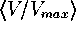-test.

We conventionally assume a flat Universe so that, with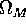and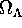being fractional contributions of matter and cosmological constant term (Carroll, Press & Turner 1992). The present value of the Hubble constant is assumed to be 75 km/s/Mpc. The GRB were considered as standard candles with a proper luminosity L and have a power-law spectrumwith a spectral index s=1.5 (Schaefer et al. 1992). Briefly, the count rate at the detector from a source at a redshift z iswhere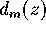is a metric distance (see Carroll et al. 1992). The number of events, N(>C), with an observed count rate exceeding C is thus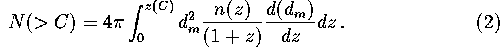The source evolution n(z) can easily be obtained using the evolutionary Green function:and the dependence t(z) for a particular cosmological model.

Fig.shows the "Green functions" for the double compact binary merging rate evolution assuming no collapse anisotropy. A very strong early evolution with time is seen; however, even for an elliptical galaxy the NS+NS merging rate is 1 per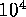yrs for an age ofyrs. The non-monotonic character of the merging rate evolution is due to different contributions of different type binaries (by initial masses, separations and types of the first mass exchange); as the detailed shape is of less importance for us now, we postpone discussing these interesting features in a separate paper. We should, however, note that a small decrease of the coalescence rate observed at the age of about 4 billion years is statistically significant and is caused by contributions of evolutionary different types of NS+NS binaries (they come from different ranges of the initial mass ratios and semimajor axes). This feature can produce a notable decrease in the-curve slope at the corresponding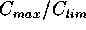. Thus, if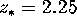the drop in sources production rate after 4 billion years from the beginning corresponds (for the flat Universe), somewhat surprisingly, just to the feature visually seen in both 2d and 3d BATSE catalog-curves; however, it would be prematurely to take the apparent feature seriously due to its low statistical significance. Another possible way that could lead to arising diverse peculiarities in the-distribution induced by the sources evolution is connected with a possible non-monotonic character of the star formation rate in galaxies.

For aspiral galaxy with constant star formation rate our calculations give a binary NS coalescence rate of the order of one per five thousand years only slightly depending on the initial mass ratio spectrum and assumptions about massive core collapse anisotropy. This rate is close to the most ``optimistic'' estimates based on evolutionary considerations (Lipunov et al 1987, 1995; Tutukov & Yungelson 1992; van den Heuvel 1994). In our calculations we used a smoothed evolutionary function assuming the star formation to occur during the first 500 million years in elliptical galaxies, and constant in spiral galaxies. In fact, the results proved to be only weakly sensitive to the smoothening over time provided that it is less than 1 billlion years (the width of the wide peak of the Green function; see Fig.).

Our theoretical models depend on three unknown parameters,,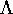,, as well as on s. The calculated-were compared with the BATSE data by using Mises-Smirnov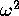test (which gives essentially the same results as Kolmogorov-Smirnov test, but uses a more smooth criterion for comparing observed and tested distribution functions; see Bol'shev & Smirnov 1965). Fig.,,show two-dimensional cuts through the parameter space. All three cuts show contour lines for confidence level according totest higher than 90%, with the maximum level being at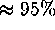in all planes.

The calculated-distributions are plotted in Fig.for different star formation starting times. Other parameters are fixed at the best-fit values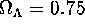and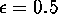. The most prominent feature of the obtained theoretical-is a notable sharp increase at low count rates due to evolutionary effects. In terms of-test, such a turnup of the-curve would lead to a sharp increase of the averagevalues when weaker sources (i.e. farther sample limits) are taken into account (Fig.). The curves turn out to be most sensitive to the parameterand depend only slightly on other parameters. We also note that a flatter GRB spectral index s=1 would favour earlier initial star formation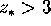and changes the-curves stronger at lower.

If the cosmological binary NS coalescences do underly the GRB phenomenon, late epochs of the initial star formation in galaxies () would yield inconsistency with the already existing BATSE data.Next: Conclusions Up: Evolution of the double Previous: Calculation of binary NS

Mike E. Prokhorov
Tue Aug 20 18:36:42 MSD 1996